# The Real Number System Real Numbers Real numbers

• Slides: 15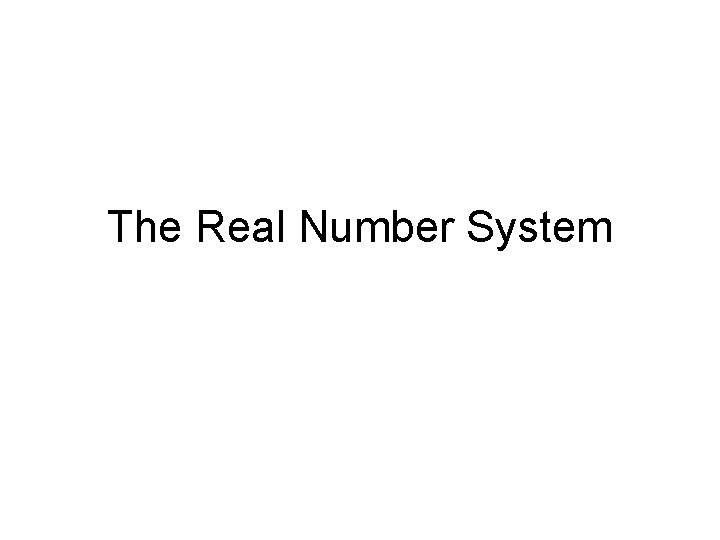The Real Number System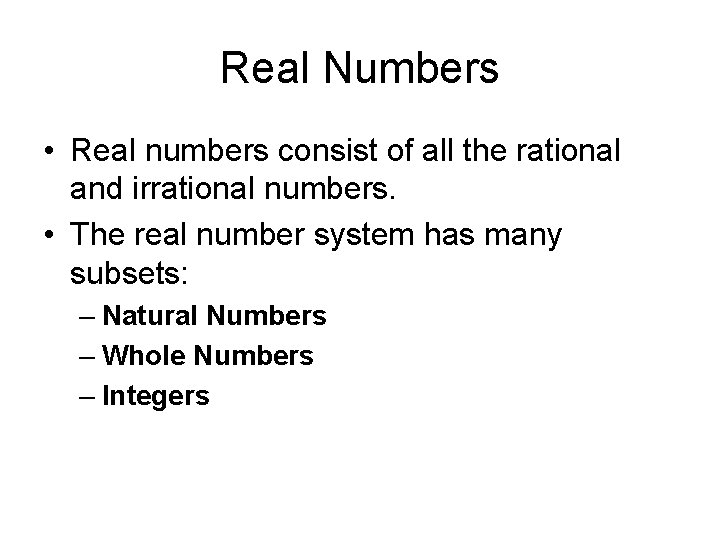Real Numbers • Real numbers consist of all the rational and irrational numbers. • The real number system has many subsets: – Natural Numbers – Whole Numbers – Integers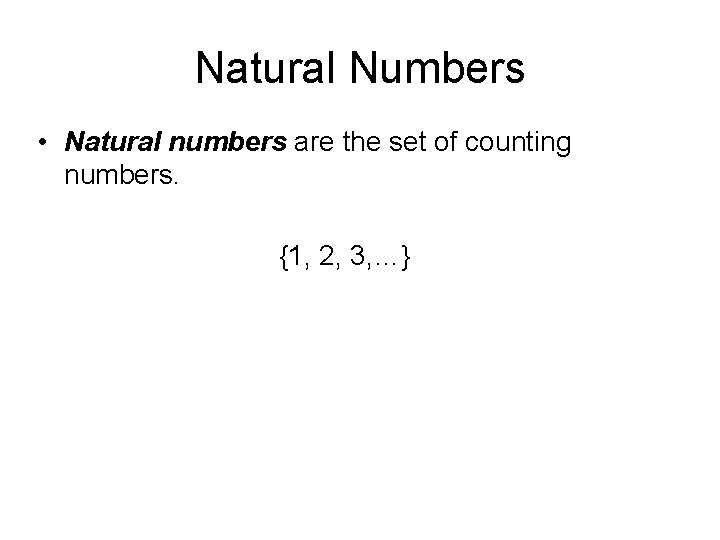Natural Numbers • Natural numbers are the set of counting numbers. {1, 2, 3, …}Whole Numbers • Whole numbers are the set of numbers that include 0 plus the set of natural numbers. {0, 1, 2, 3, 4, 5, …}Integers • Integers are the set of whole numbers and their opposites. {…, -3, -2, -1, 0, 1, 2, 3, …}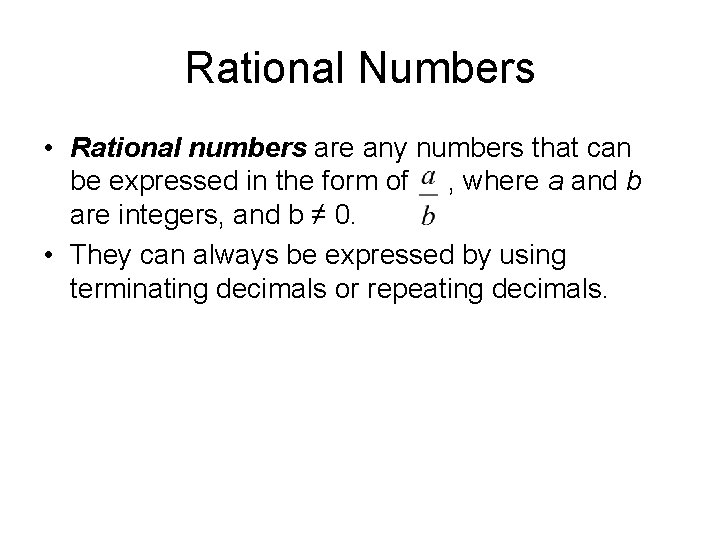Rational Numbers • Rational numbers are any numbers that can be expressed in the form of , where a and b are integers, and b ≠ 0. • They can always be expressed by using terminating decimals or repeating decimals.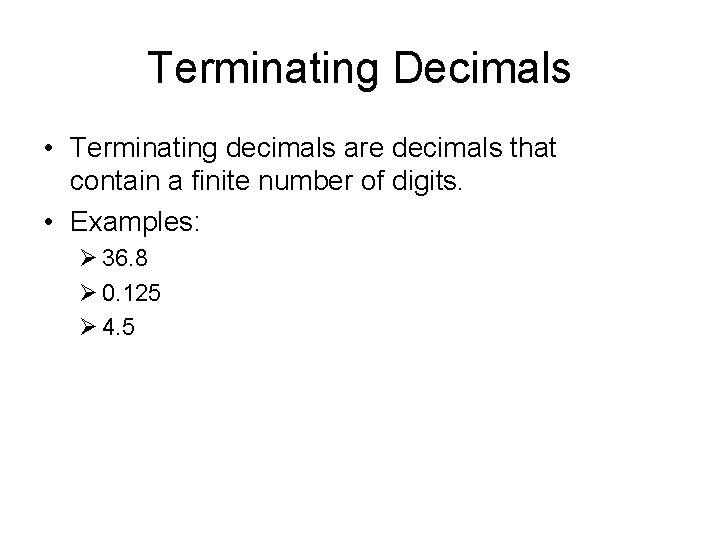Terminating Decimals • Terminating decimals are decimals that contain a finite number of digits. • Examples: Ø 36. 8 Ø 0. 125 Ø 4. 5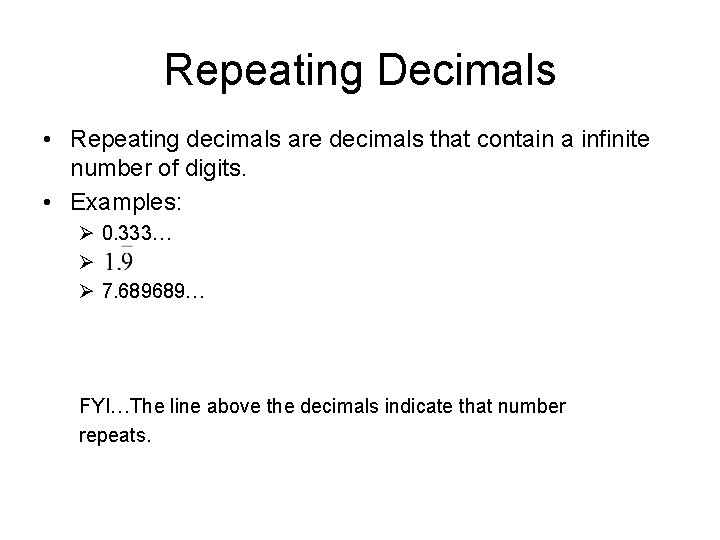Repeating Decimals • Repeating decimals are decimals that contain a infinite number of digits. • Examples: Ø 0. 333… Ø Ø 7. 689689… FYI…The line above the decimals indicate that number repeats.Irrational Numbers • Irrational numbers are any numbers that cannot be expressed as. • They are expressed as non-terminating, nonrepeating decimals; decimals that go on forever without repeating a pattern. • Examples of irrational numbers: – 0. 3433433334… – 45. 86745893… – (pi) –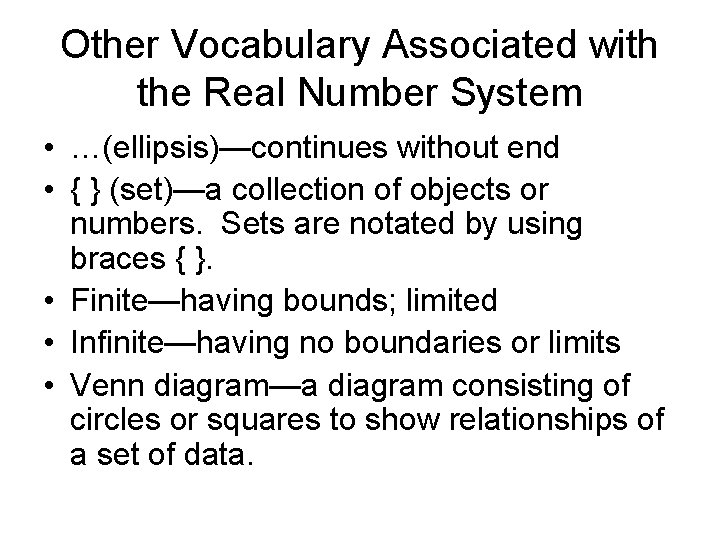Other Vocabulary Associated with the Real Number System • …(ellipsis)—continues without end • { } (set)—a collection of objects or numbers. Sets are notated by using braces { }. • Finite—having bounds; limited • Infinite—having no boundaries or limits • Venn diagram—a diagram consisting of circles or squares to show relationships of a set of data.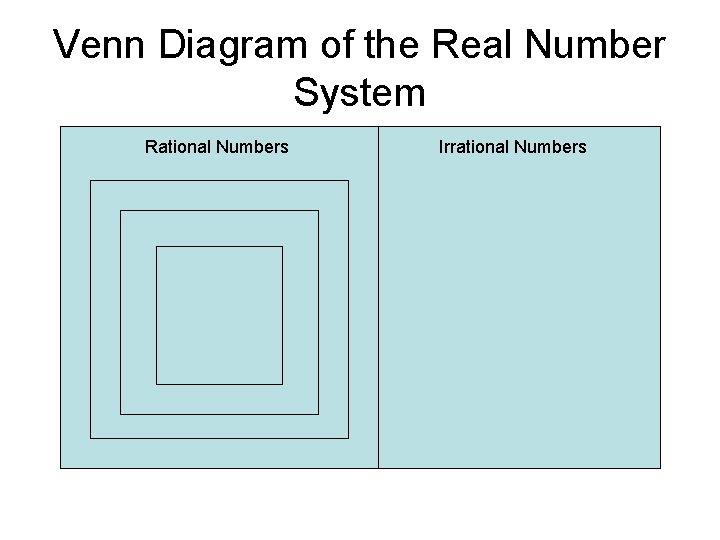Venn Diagram of the Real Number System Rational Numbers Irrational NumbersExample • Classify all the following numbers as natural, whole, integer, rational, or irrational. List all that apply. a. 117 b. 0 c. -12. 64039… d. -½ e. 6. 36 f. g. -3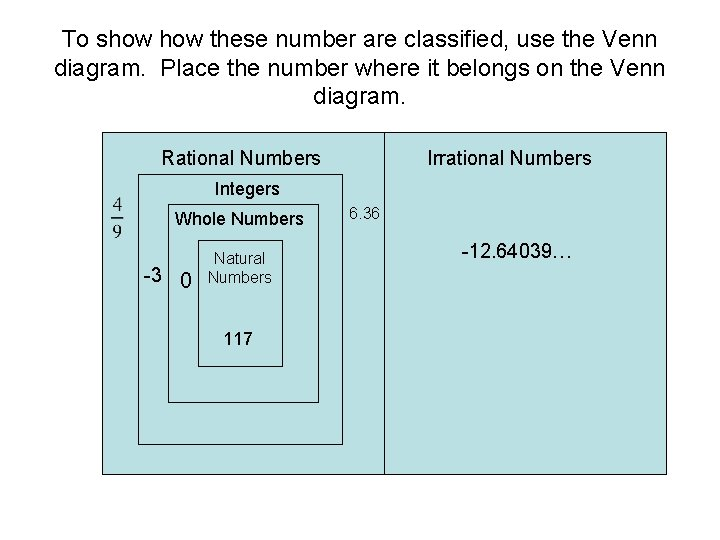To show these number are classified, use the Venn diagram. Place the number where it belongs on the Venn diagram. Rational Numbers Irrational Numbers Integers Whole Numbers -3 0 Natural Numbers 117 6. 36 -12. 64039…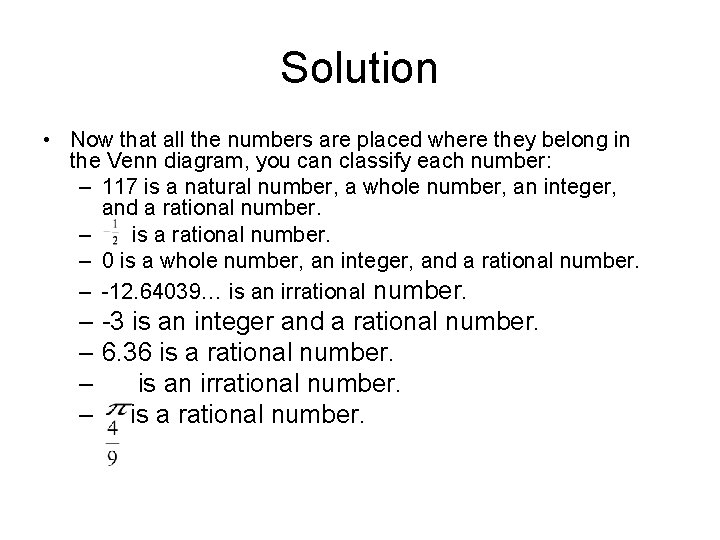Solution • Now that all the numbers are placed where they belong in the Venn diagram, you can classify each number: – 117 is a natural number, a whole number, an integer, and a rational number. – is a rational number. – 0 is a whole number, an integer, and a rational number. – -12. 64039… is an irrational number. – -3 is an integer and a rational number. – 6. 36 is a rational number. – is an irrational number. – is a rational number.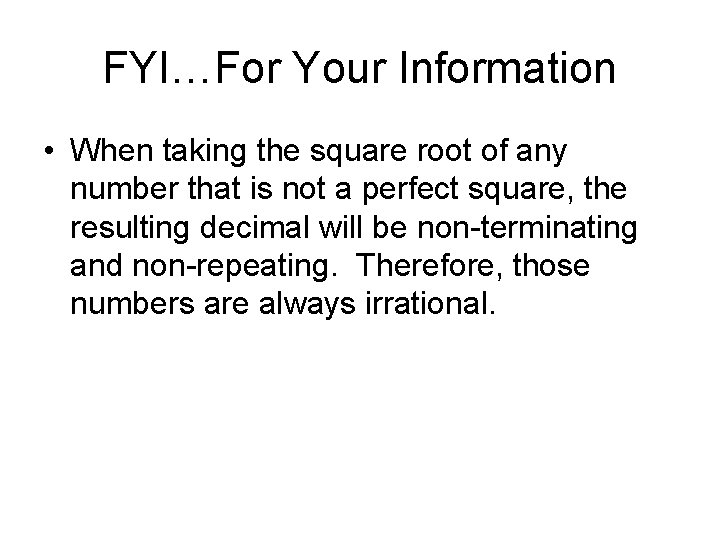FYI…For Your Information • When taking the square root of any number that is not a perfect square, the resulting decimal will be non-terminating and non-repeating. Therefore, those numbers are always irrational.